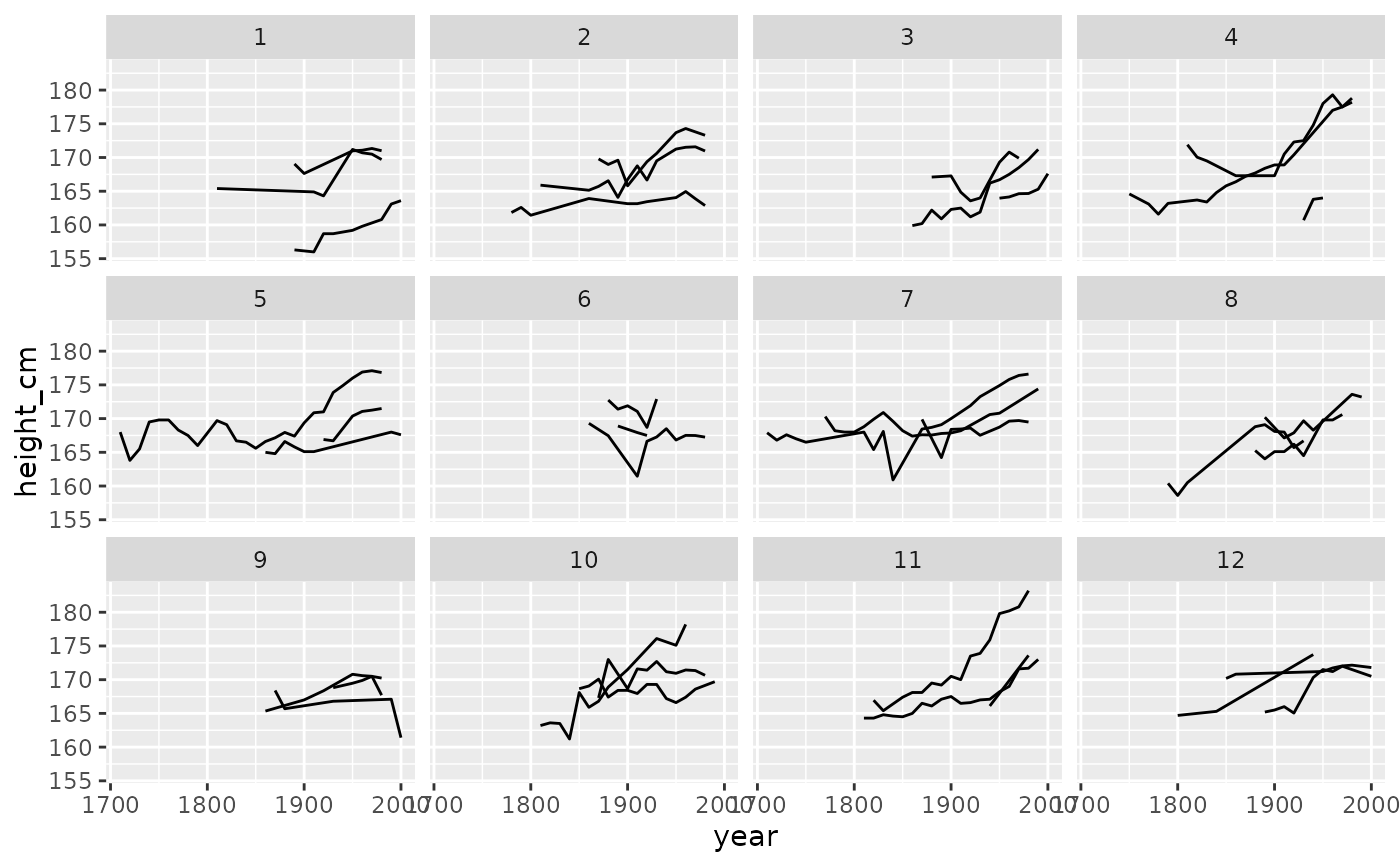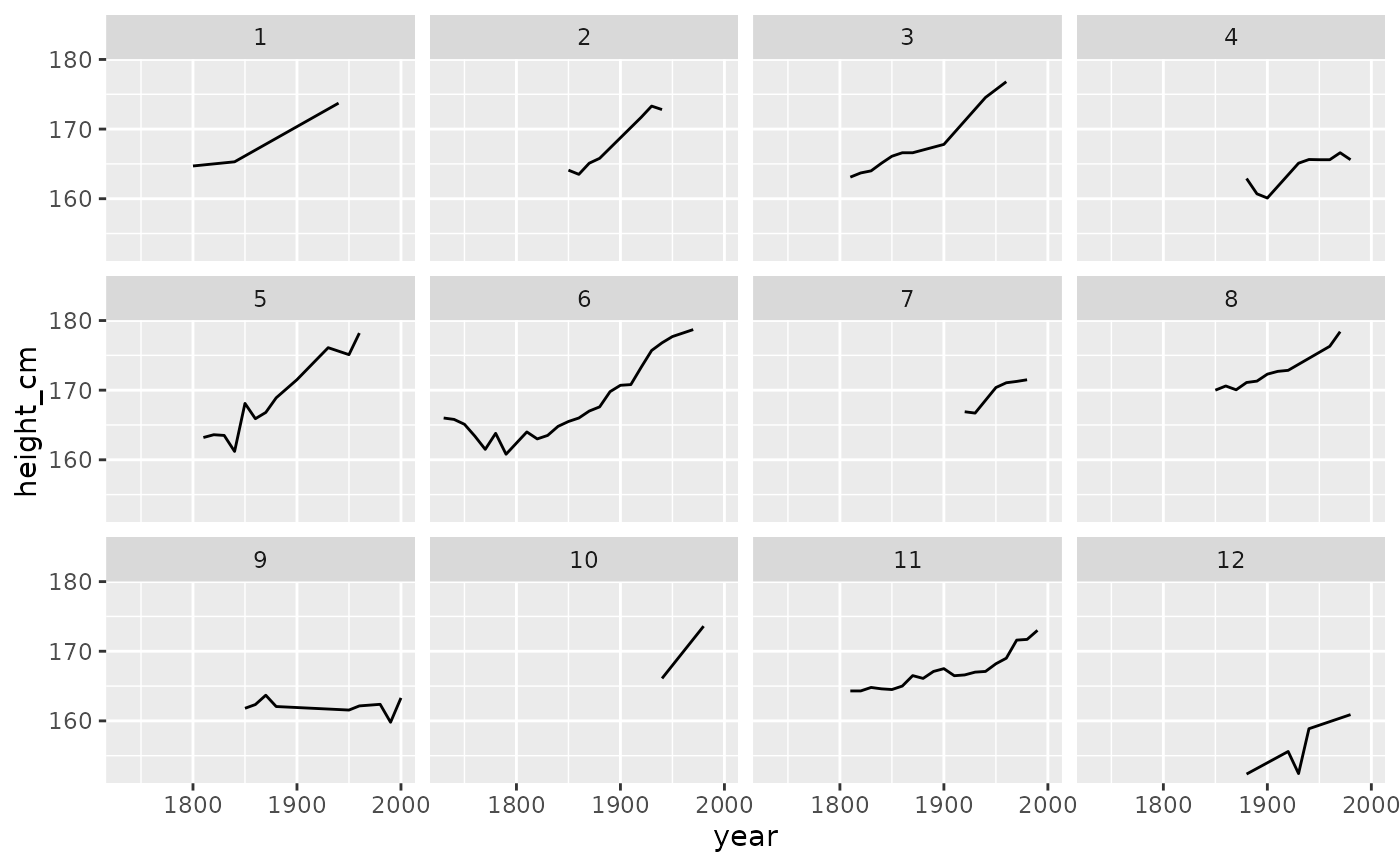This function requires a tbl_ts object, which can be created with tsibble::as_tsibble(). Under the hood, facet_strata is powered by stratify_keys() and sample_n_keys().

## Usage

facet_sample(
n_per_facet = 3,
n_facets = 12,
nrow = NULL,
ncol = NULL,
scales = "fixed",
shrink = TRUE,
strip.position = "top"
)

## Arguments

n_per_facet

Number of keys per facet you want to plot. Default is 3.

n_facets

Number of facets to create. Default is 12

nrow, ncol

Number of rows and columns.

scales

Should scales be fixed ("fixed", the default), free ("free"), or free in one dimension ("free_x", "free_y")?

shrink

If TRUE, will shrink scales to fit output of statistics, not raw data. If FALSE, will be range of raw data before statistical summary.

strip.position

By default, the labels are displayed on the top of the plot. Using strip.position it is possible to place the labels on either of the four sides by setting strip.position = c("top", "bottom", "left", "right")

a ggplot object

## Examples

library(ggplot2)
ggplot(heights,
aes(x = year,
y = height_cm,
group = country)) +
geom_line() +
facet_sample()ggplot(heights,
aes(x = year,
y = height_cm,
group = country)) +
geom_line() +
facet_sample(n_per_facet = 1,
n_facets = 12)